## 13.1 视觉皮层机理

David H. Hubel和Torsten Wiesel于1958、1959年在猫的身上做实验，给出了关于视觉皮层结构的深刻见解（作者因此与1981年获得诺贝尔生物或医学奖）。特别的，他们指出视觉皮层的许多神经元具有一个很小的局部接受域（local receptive field）。也就是说，这种神经元只会对视野里一个有限的区域做出反应（如图13-1所示，这5个神经元的局部接受域由5个虚线表示）。不同神经元的局部接受与可能部分重叠，放在一起就构成了整个视野。此外，作者指出一些神经元只对水平线感兴趣，而另一些只对线的不同方向做出反应。他们也注意到，一些神经元具有较大的接受域，只会对由低级模式组合成的高级模式做出反应。这些观察结果带来了这样一个理念：高级神经元只以临近的低级神经元为基础（如图13-1，高层神经元只与部分地层神经元连接）。这一强大的结构可以检测到视觉领域的各种复杂模式。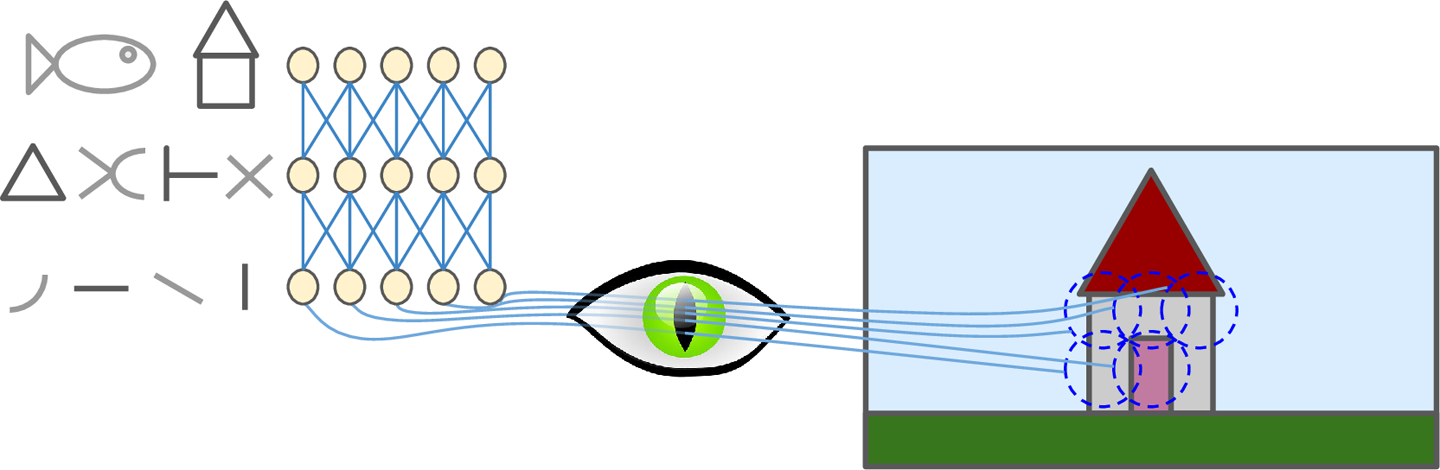## 13.2 卷积层

CNN最重要的构件就是卷积层（卷积是一种数学运算，度量两个函数乘积的逐点积分。其与傅里叶变换与拉普拉斯变换高度相关，在信号处理中应用广泛。卷积层实际上使用了互相关，这与卷积是相似的。卷积处理的是连续变量，互相关好像是离散变量。）：第一个卷积层的神经元并不和所有的输入全连接，只与其接受域的像素点连接（如图13-2）。依次的，第二个卷积层也与第一个卷积层部分相连。这一架构使得第一个隐层专注于低级别特征，然后在随后的隐层中将低级别特征进行组合。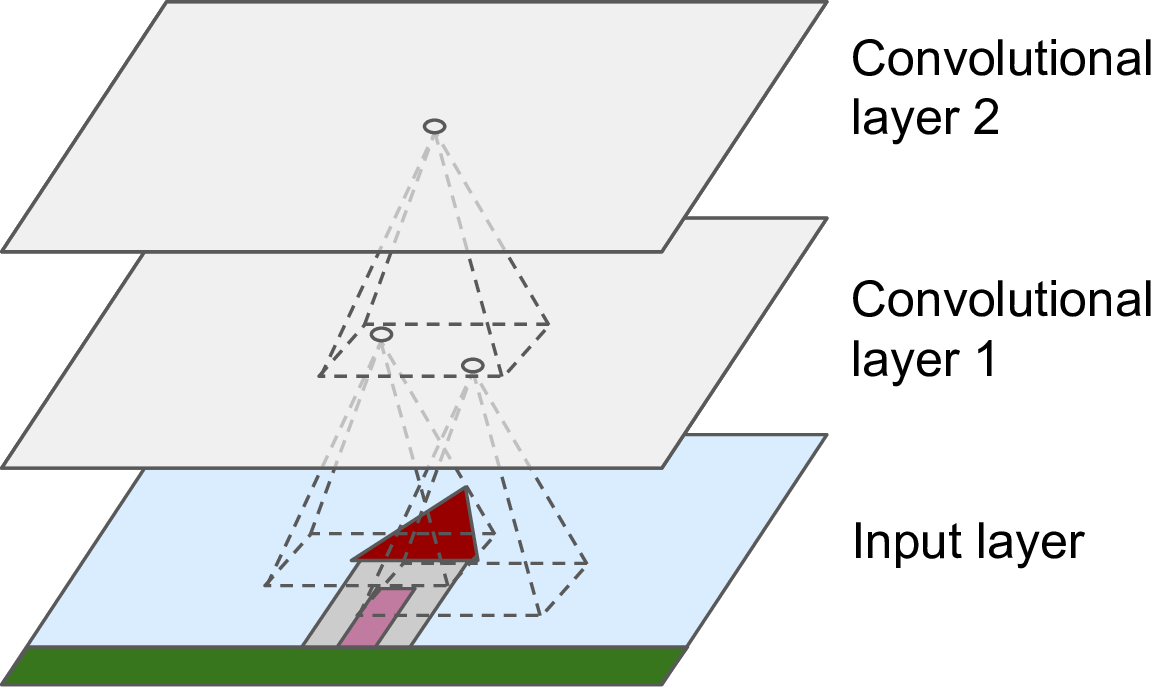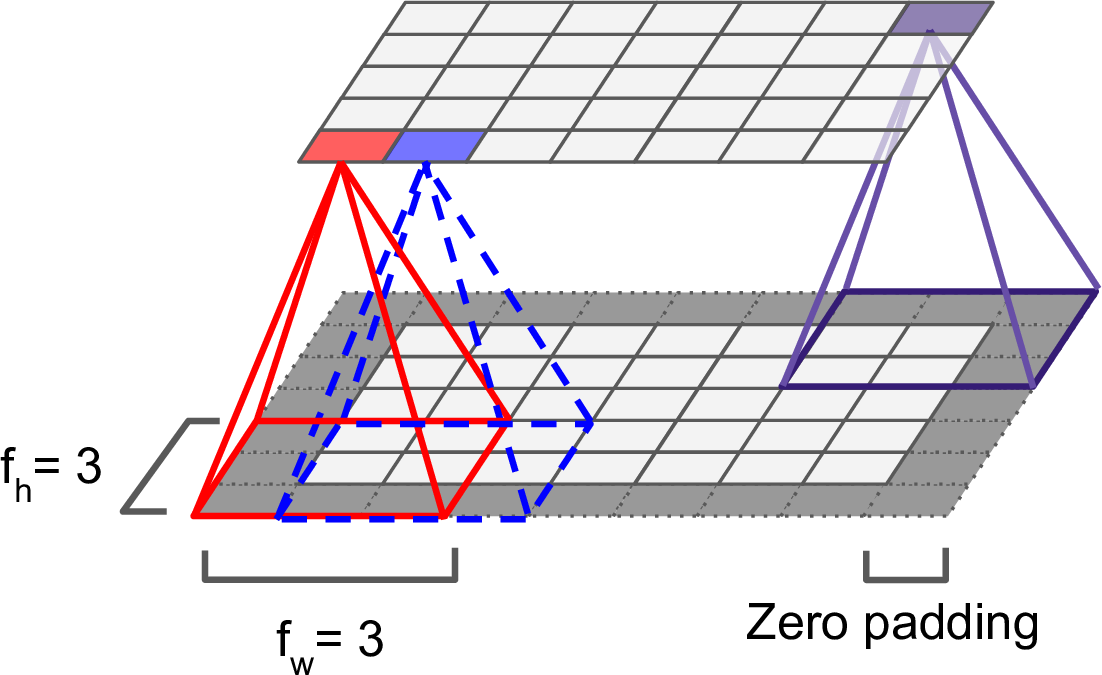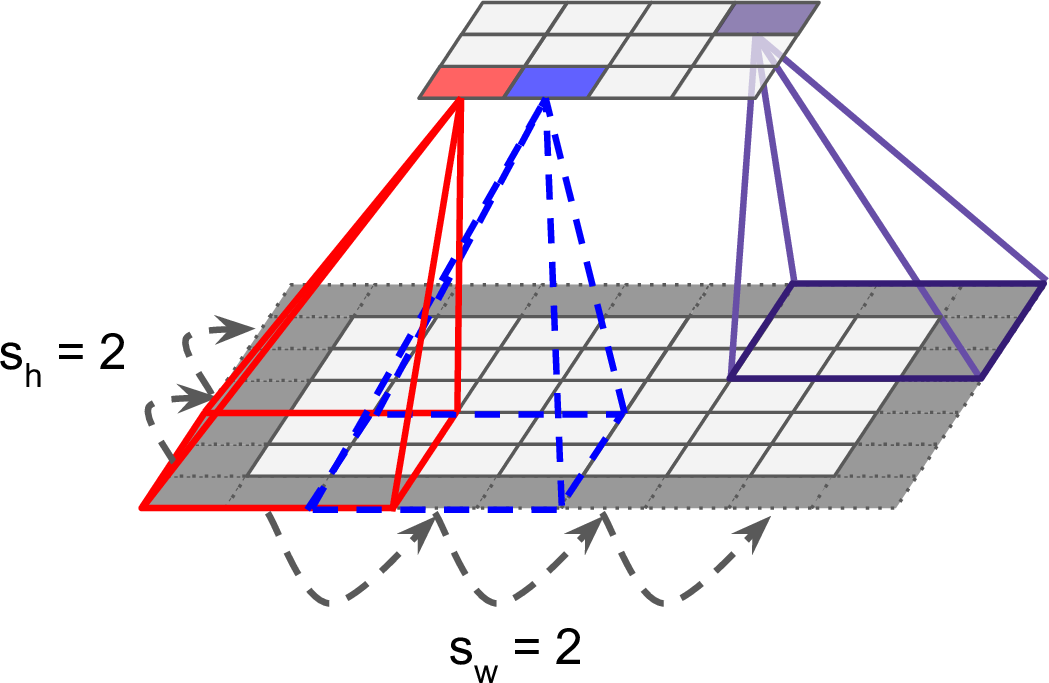### 13.2.1 过滤器

神经元的权重可以表示接受域内的一个小的影像。例如，图13-5展示了两套可能的权重，称作过滤器（或者卷积核）。第一个过滤器描绘了一个在正中间拥有白色直线的黑块（这是一个$7 \times 7$的矩阵，除了中间那条竖线是1之外，其他地方都是0）。神经元使用这样的权重，只会注意到其接受域中间的那条竖线，而忽视其他像素点。第二个过滤器是同样大小的黑块，但中间是条白色的水平线。神经元使用这样的权重，只会注意到其接受域中间的那条直线。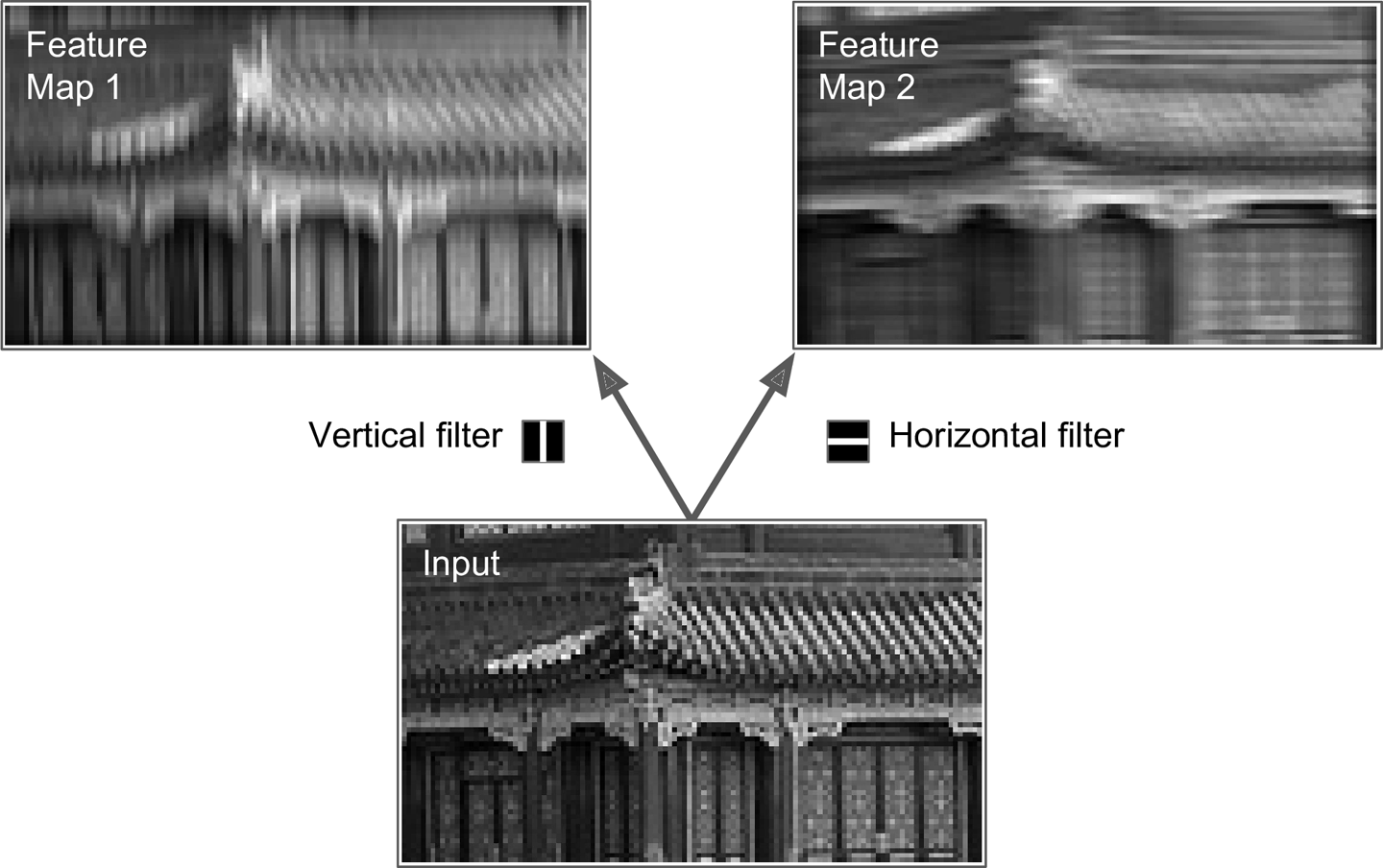### 13.2.2 堆叠多重特征图（Stacking Multiple Feature Maps）\begin{align*}
z_{i,j,k} = b_k + \sum_{u=1}^{f_h}\sum_{v=1}^{f_w}\sum_{k'=1}^{f_{n'}} x_{i',j',k'} \cdot w_{u,v,k',k}
\end{align*}

• $i' = u \cdot s_w + f_w - 1$
• $j' = v \cdot s_h + f_h - 1$
• $z_{i,j,k}$是第$l$卷积层第$k$个特征图，第$i$行$j$列神经元的输出。
• $s_h$和$s_w$分别是垂直和水平跨度，$f_h$和$f_w$分别是接受域的高和宽，$f_{n'}$是前层特征图的个数（$l-1$层）。
• $x_{i',j',k'}$是第$l-1$层，第$k'$个特征图（如果前层是输入层，那就是第$k'$个通道），第$i'$行$j'$列神经元的输出。
• $b_k$是第$l$层第$k$个特征图的偏置项。可以把它看做一个开关，来调整第$k$个特征图的整体亮度。
• $w_{u,v,k',k}$是第$l$层第$k$个特征图中的任意一个神经元，它的第$k'$个特征图的第$u$行第$v$列的输入的权重。

### 13.2.3 TensorFlow实现

import numpy as np
from sklearn.datasets import load_sample_images

# 加载两个图片
dataset = np.array(load_sample_images().images, dtype=np.float32)
batch_size, height, width, channels = dataset.shape

# 创建两个过滤器
filters_test = np.zeros(shape=(7, 7, channels, 2), dtype=np.float32)
filters_test[:, 3, :, 0] = 1 # 垂线
filters_test[3, :, :, 1] = 1 # 水平线

# 创建一个图，包括输入X和使用了两个过滤器的一个卷积层。
X = tf.placeholder(tf.float32, shape=(None, height, width, channels))
convolution = tf.nn.conv2d(X, filters, strides=[1,2,2,1], padding="SAME")

with tf.Session() as sess:
output = sess.run(convolution, feed_dict={X: dataset})

plt.imshow(output[0, :, :, 1]) # 绘制第一幅图的第二个特征图
plt.show()　　

• X是mini-batch输入（一个4D张量，如前所示）
• filters是一个过滤器集（4D张量，如前所示）
• strides拥有4个元素的一维数组，中间两个元素表示垂直和水平跨度。第一和第四个元素目前直接设置为1。以后可能会用来表示批跨度（跳过一些实例），以及通道跨度（跳过先前层的一些通道或者特征图）。
• padding有"VALID"、"SAME"两个选择：
• 如果设置为"VALID"，卷积层不使用0填充，可能会直接忽略边缘的一些输入，如图13-7所示（为简单起见，图中只显示了中间的水平线）。
• 如果设置为"SAME"，卷积层在必要的时候使用0填充。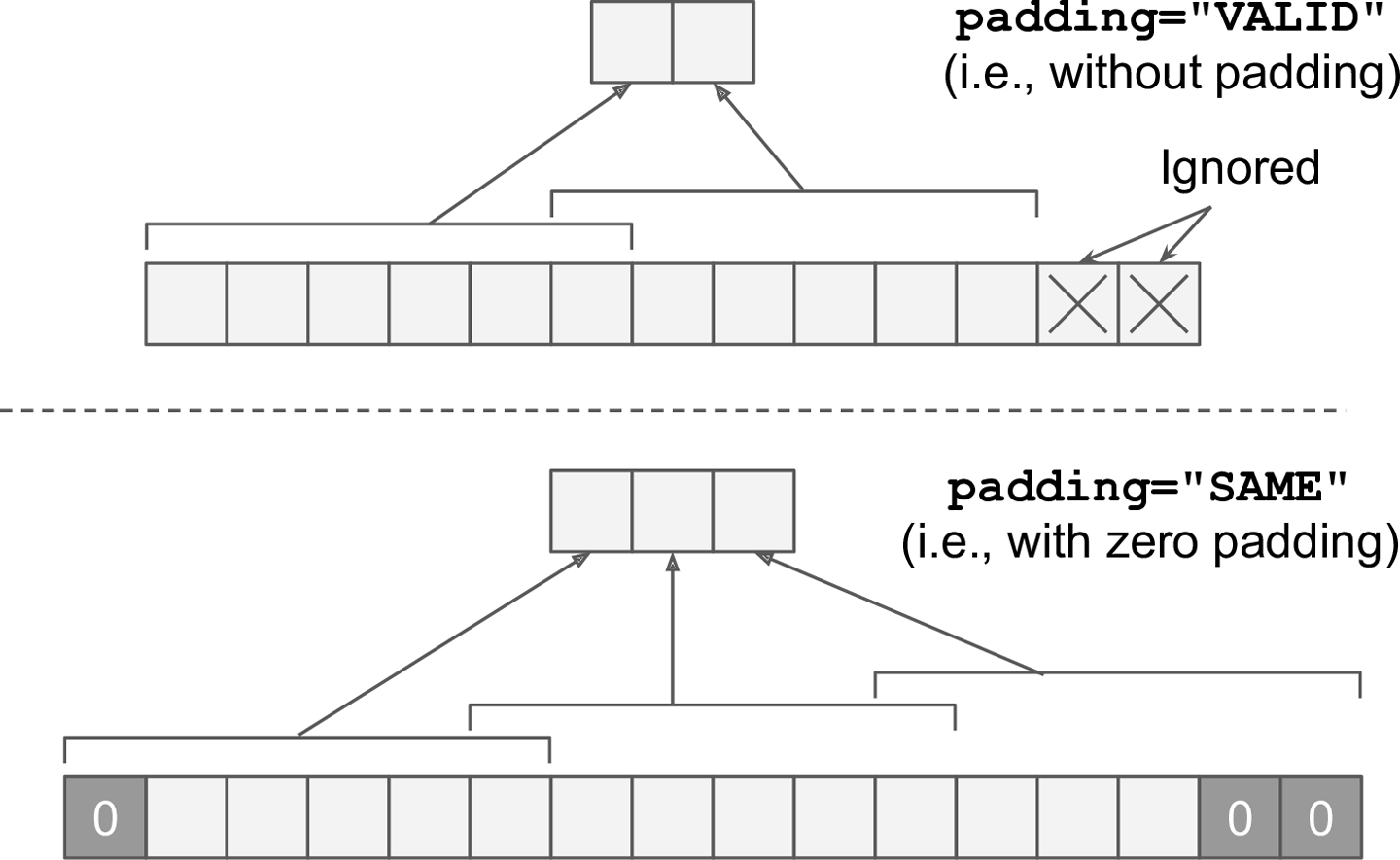### 13.2.3 内存要求

CNN的另一个问题是卷积层需要使用大量的RAM，尤其是训练的时候，因为反向传播需要前向传递的所有中间值。

## 13.3 池化层（Pooling Layer）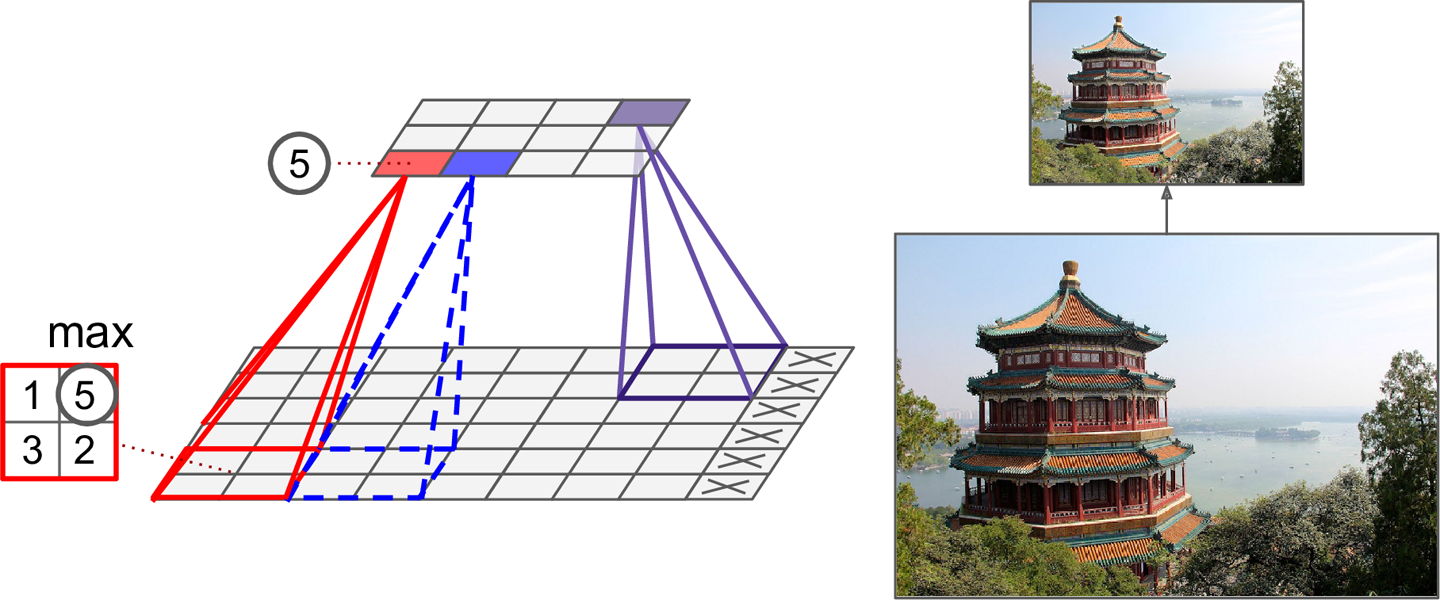TensorFlow实现：

[...] # load the image dataset, just like above

# Create a graph with input X plus a max pooling layer
X = tf.placeholder(tf.float32, shape=(None, height, width, channels))
max_pool = tf.nn.max_pool(X, ksize=[1,2,2,1], strides=[1,2,2,1],padding="VALID")

with tf.Session() as sess:
output = sess.run(max_pool, feed_dict={X: dataset})

plt.imshow(output.astype(np.uint8)) # plot the output for the 1st image
plt.show()


## 13.4 CNN架构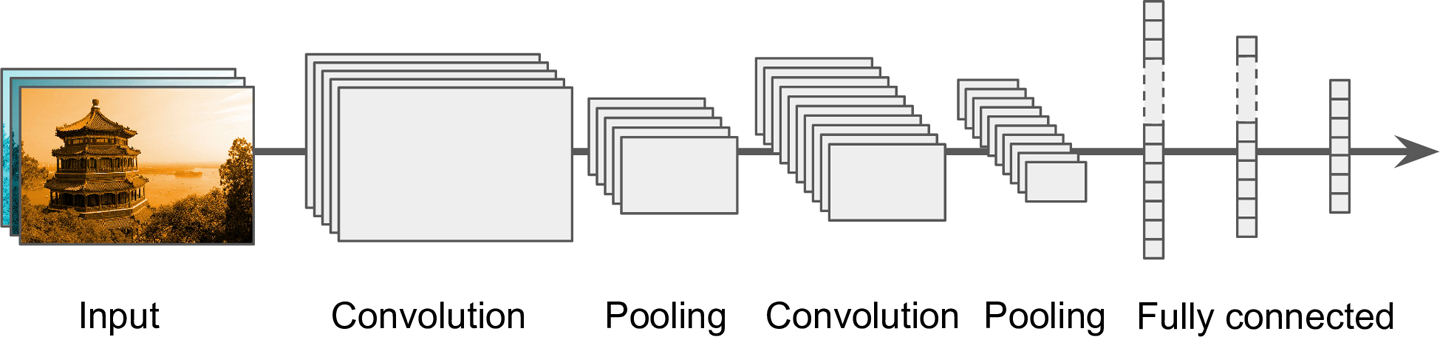### 13.4.1 LeNet-5

LeNet-5是最广为人知的CNN架构。在1998年提出比广泛应用于手写数字识别（MNIST）。由以下各层组成：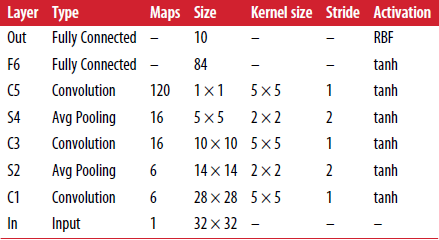• MNIST图像是28 × 28像素，0填充后是32 × 32像素，并且在输入之前进行标准化（normalized）。剩下的各层不再填充。
• 这里的平均池化层（average pooling layers）比常规的稍微复杂：每个神经元求出其输入均值后，要对该均值乘以一个可学习的系数（每个特征图一个系数）并加上一个可学习的偏置（也是每个特征图一个），然后将结果应用于激活函数。
• C3中的大部分神经元只与S2中的3到4个特征图连接，而不是全部的6个。
• 输出层也有一点特殊，具体可以看论文。

LeNet-5数字分类的demo，可参考Yann LeCun的网站（"LENET" 章节）。

posted on 2018-05-23 17:57  royhoo  阅读(3391)  评论(2编辑  收藏  举报